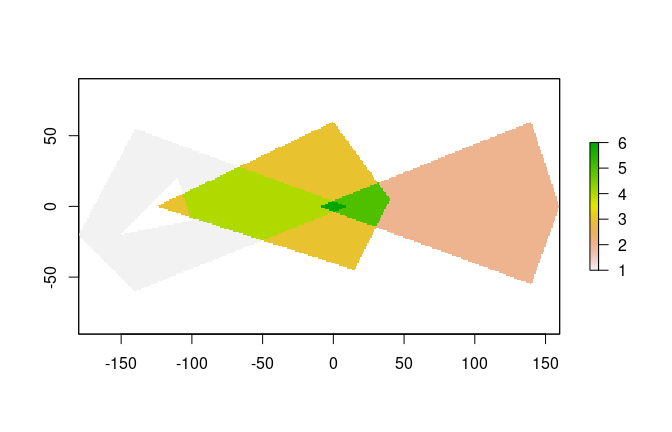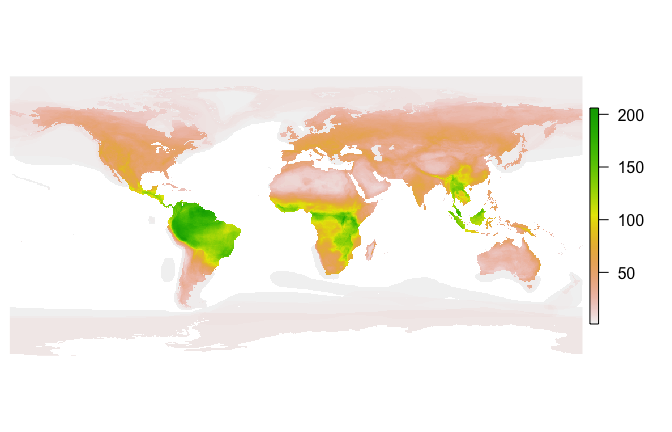# Using Fasterize

#### 2022-12-07

fasterize is a high-performance replacement for the rasterize() function in the raster package.

Functionality is currently limited to rasterizing polygons in sf-type data frames.

## Installation

Install the current version of fasterize from CRAN:

install.packages('fasterize')

Install the development version of fasterize with devtools:

devtools::install_github("ecohealthalliance/fasterize")

fasterize uses Rcpp and thus requires a compile toolchain to install from source. Testing (and most use) requires sf, which requires GDAL (>= 2.0.0), GEOS (>= 3.3.0), and PROJ.4 (>= 4.8.0) to be installed on your system.

## Usage

The main function, fasterize(), takes the same inputs as raster::rasterize() but currently has fewer options and is is limited to rasterizing polygons.

A method for creating empty rasters from sf objects is provided, and raster plot methods are re-exported.

library(raster)
library(fasterize)
library(sf)
p1 <- rbind(c(-180,-20), c(-140,55), c(10, 0), c(-140,-60), c(-180,-20))
hole <- rbind(c(-150,-20), c(-100,-10), c(-110,20), c(-150,-20))
p1 <- list(p1, hole)
p2 <- list(rbind(c(-10,0), c(140,60), c(160,0), c(140,-55), c(-10,0)))
p3 <- list(rbind(c(-125,0), c(0,60), c(40,5), c(15,-45), c(-125,0)))
pols <- st_sf(value = c(1,2,3),
geometry = st_sfc(lapply(list(p1, p2, p3), st_polygon)))
r <- raster(pols, res = 1)
r <- fasterize(pols, r, field = "value", fun="sum")
plot(r)## Performance

Let’s compare fasterize() to raster::rasterize():

pols_r <- as(pols, "Spatial")
bench <- microbenchmark::microbenchmark(
rasterize = r <- raster::rasterize(pols_r, r, field = "value", fun="sum"),
fasterize = f <- fasterize(pols, r, field = "value", fun="sum"),
unit = "ms"
)
print(bench, digits = 3)
#> Unit: milliseconds
#>       expr     min     lq    mean  median     uq    max neval cld
#>  rasterize 340.376 359.69 379.197 369.552 386.65 497.83   100   b
#>  fasterize   0.337   0.37   0.545   0.414   0.64   2.51   100  a

Note that even with terra, it’s still faster.

pols_v <- terra::vect(pols_r)
rt <- terra::rast(r)
bench <- microbenchmark::microbenchmark(
terra_rasterize = r0 <- terra::rasterize(pols_v, rt, field = "value", fun="sum"),
fasterize = f <- fasterize(pols, r, field = "value", fun="sum"),
unit = "ms"
)
print(bench, digits = 3)

#> Unit: milliseconds #> expr min lq mean median uq max neval cld #> rasterize 6.148 6.939 7.66 7.22 7.45 50.5 100 b #> fasterize 0.875 0.931 1.19 0.97 1.00 21.4 100 a

We can do even better if we don’t materialize the pixel values and simply use the created index in efficient ways - but currently, we have to actually allocate the entire raster so there’s a simple memory limit there (and we’re always using double floating point type). Please see the Issues on the project respository and the CONTRIBUTING.md if you are interested.

How does fasterize() do on a large set of polygons? Here I download the IUCN shapefile for the ranges of all terrestrial mammals and generate a 1/6 degree world map of mammalian biodiversity by rasterizing all the layers.

if(!dir.exists("Mammals_Terrestrial")) {
"https://s3.amazonaws.com/hp3-shapefiles/Mammals_Terrestrial.zip",
destfile = "Mammals_Terrestrial.zip") # <-- 383 MB
unzip("Mammals_Terrestrial.zip", exdir = ".")
}
mammal_shapes <- st_read("Mammals_Terrestrial")
#> Reading layer Mammals_Terrestrial' from data source /Users/noamross/dropbox-eha/projects-eha/fasterize/Mammals_Terrestrial' using driver ESRI Shapefile'
#> Simple feature collection with 42714 features and 27 fields
#> geometry type:  MULTIPOLYGON
#> dimension:      XY
#> bbox:           xmin: -180 ymin: -85.58276 xmax: 180 ymax: 89.99999
#> epsg (SRID):    4326
#> proj4string:    +proj=longlat +datum=WGS84 +no_defs
mammal_raster <- raster(mammal_shapes, res = 1/6)
bench2 <- microbenchmark::microbenchmark(
mammals = mammal_raster <- fasterize(mammal_shapes, mammal_raster, fun="sum"),
times=20, unit = "s")
print(bench2, digits=3)
#> Unit: seconds
#>     expr   min    lq  mean median    uq   max neval
#>  mammals 0.856 0.869 0.887   0.88 0.902 0.955    20
par(mar=c(0,0.5,0,0.5))
plot(mammal_raster, axes=FALSE, box=FALSE)`